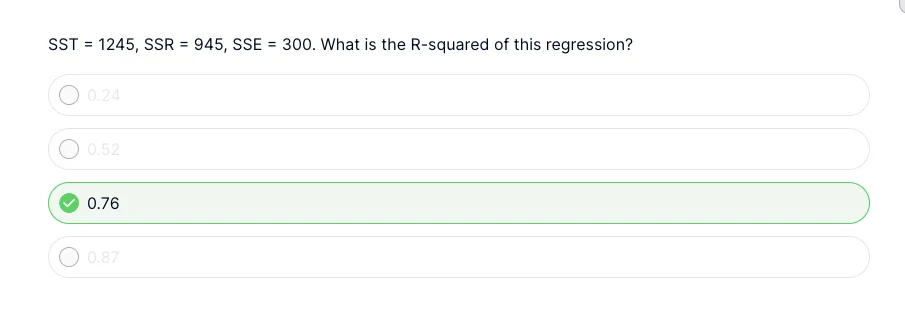03 Jan 2023

Posted on:

31 Dec 2022

0

# Calculations required

How is this calculated?Instructor
Posted on:

03 Jan 2023

1

Hey Anita,

Thank you for reaching out.

As stated in the lecture preceding the quiz, the R-squared is calculating by dividing the SSR by the SST. Therefore, dividing 945 by 1245 gives the correct answer.

Kind regards,
365 Hristina